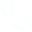# Python | Interprocess Communication

## interprocess communication

Interprocess communication - Queue
from multiprocessing import Queue
q=Queue(3) #Initialize a Queue object, which can receive up to three put messages
q.put("Message 1")
q.put("Message 2")
print(q.full()) #False
q.put("Message 3")
print(q.full()) #True

#Because the message queue is full, the following try will throw an exception, the first try will wait 2 seconds before throwing an exception, and the second try will throw an exception immediately
try:
q.put("Message 4",True,2)
except:
print("The message queue is full, the number of existing messages: %s"%q.qsize())

try:
q.put_nowait("Message 4")
except:
print("The message queue is full, the number of existing messages: %s"%q.qsize())

#Recommended method, first determine whether the message queue is full, and then write
if not q.full():
q.put_nowait("Message 4")

#When reading a message, first determine whether the message queue is empty, and then read
if not q.empty():
for i in range(q.qsize()):
print(q.get_nowait())
illustrate:
When initializing the Queue() object (for example: q=Queue()), if the maximum number of messages that can be received is not specified in the parentheses, or the number is negative, then there is no upper limit on the number of acceptable messages (until the end of memory) ;

Queue.qsize(): Returns the number of messages contained in the current queue;
Queue.empty(): If the queue is empty, return True, otherwise False;
Queue.full(): If the queue is full, return True, otherwise False;
Queue.get([block[, timeout]]): Get a message in the queue, and then remove it from the queue, the default value of block is True;
1) If the default value of block is used and the timeout (in seconds) is not set, if the message queue is empty, the program will be blocked (stop in the reading state) until the message is read from the message queue, if the timeout is set , it will wait for timeout seconds, and if no message has been read, a "Queue.Empty" exception will be thrown;
2) If the block value is False, if the message queue is empty, the "Queue.Empty" exception will be thrown immediately;

Queue.get_nowait(): Equivalent to Queue.get(False);
Queue.put(item,[block[, timeout]]): Write the item message to the queue, the default value of block is True;
1) If the default value of block is used and the timeout (in seconds) is not set, if the message queue has no space to write, the program will be blocked (stop in the writing state) until space is vacated from the message queue. If timeout is set, it will wait for timeout seconds, and if there is no space, a "Queue.Full" exception will be thrown;

2) If the block value is False, if there is no space to write in the message queue, the "Queue.Full" exception will be thrown immediately;

Queue.put_nowait(item): Equivalent to Queue.put(item, False);
Example:

import os, multiprocessing, time

def producer(x):
for i in range(10):
time.sleep(0.5)
print('Produced +++++++pid{} {}'.format(os.getpid(), i))
x.put('pid{} {}'.format(os.getpid(), i))

def consumer(x):
for i in range(10):
time.sleep(0.3)
print('Consumed -------{}'.format(x.get()))

if __name__ == '__main__':
q = multiprocessing.Queue()

p1 = multiprocessing.Process(target=producer, args=(q,))
p2 = multiprocessing.Process(target=producer, args=(q,))
p3 = multiprocessing.Process(target=producer, args=(q,))
p1.start()
p2.start()
p3.start()

c2 = multiprocessing.Process(target=consumer, args=(q,))
c2.start()

## Related Articles

• ### A detailed explanation of Hadoop core architecture HDFS

Knowledge Base Team

• ### What Does IOT Mean

Knowledge Base Team

• ### 6 Optional Technologies for Data Storage

Knowledge Base Team

• ### What Is Blockchain Technology

Knowledge Base Team

## Explore More Special Offers

1. ### Short Message Service(SMS) & Mail Service

50,000 email package starts as low as USD 1.99, 120 short messages start at only USD 1.00Contact Us